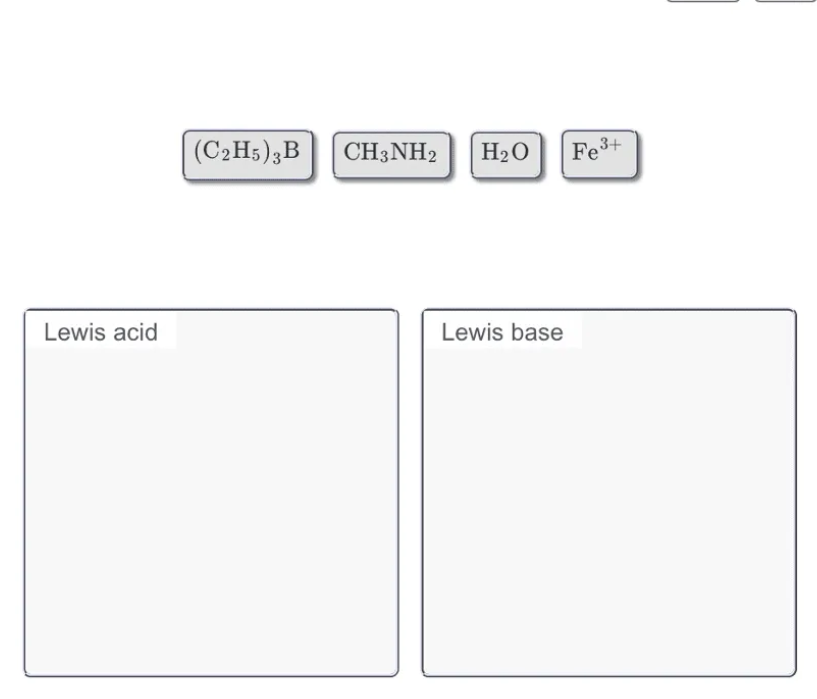# Problem: Classify each of the following as either a Lewis acid or a Lewis base. Drag the appropriate items to their respective bins.(C2H5)3BCH3NH2H2OFe3+

###### FREE Expert Solution

We’re being asked which of the given compounds is a Lewis acid or Lewis Base

Let’s first define a Lewis acid and a Lewis base.

Based on the Lewis definition:

Lewis acid is an electron pair acceptor.

Some characteristics of a Lewis acid:

When hydrogen is connected to an electronegative element such as P, O, N, S or halogens

▪ hydrogen gains a partially positive charge → makes hydrogen act as a Lewis acid

Positively charged metal ions

82% (45 ratings)###### Problem Details

Classify each of the following as either a Lewis acid or a Lewis base. Drag the appropriate items to their respective bins.

(C2H5)3B

CH3NH2

H2O

Fe3+